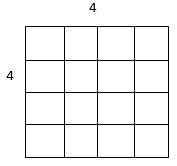# 11-Plus Exam Illustrations - Maths Quiz - Square Numbers and Square Roots (Questions)

There are many times in entrance tests that your child may need to recognise square numbers. Essentially, they are the numbers which are made by multiplying one number by itself. They are called square numbers because they can be represented as a square:The square is made by counting four along and four down; the total number of squares is sixteen. 4 x 4 = 16 or, in proper terminology, 42. This is also called 'four to the power two'

The square number series is easy to write sequencing questions for. If you ensure your child knows the series, you are sure to get an extra mark somewhere. 1 x 1 = 1, 2 x 2 = 4... This is the start of the endless list of square numbers:

 1 4 9 16 25 36 49 64 100 121 144 169 196

The cube numbers occasionally turn up as well; these are the numbers you get when you multiply a figure by itself and then by itself again. Think of the cube which is made from the 4 x 4 square shown above. Now have this as the face of a cube which has a depth of 4... The number of little cubes (as opposed to little squares which made up the big square) is 4 x 4 x 4 = 64.

The cube number series starts like this:

 1 8 27 64 125 216

There is rarely any reason to go beyond this at this stage!

Finding a square root can only really be done using trial and error. Simply pick a number that, when multiplied by itself, equals something near the figure. Then add or subtract one and multiply that number by itself until you've found a closer figure.

For example, find the square root of 441:

We know that 20 x 20 = 400 so let's try working out 21 x 21. That works out as 441 so 21 is the square root of 441.